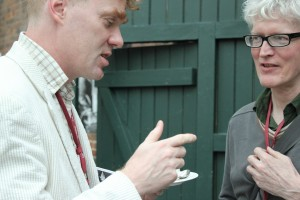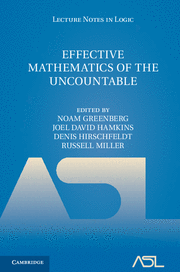# The hierarchy of equivalence relations on the natural numbers under computable reducibility, Chicheley Hall, June 2012

This will be a talk at The Incomputable, a workshop held June 12-15, 2012 at the Kavli Royal Society International Centre at Chicheley Hall as a part of the program Semantics and Syntax: A Legacy of Alan Turing organized by the Isaac Newton Institute of Mathematical Sciences in Cambridge, UK.Abstract.  I will speak on the computable analogue of the theory of equivalence relations on the reals under Borel reducibility.  The Borel theory has been enormously successful in clarifying the hierarchy of classification problems arising throughout mathematics.  The computable analogue, meanwhile, appears to be particularly well-suited for an analysis of the c.e. instances of those problems, a rich context with many natural examples, such as the isomorphism relation on c.e. graphs or on computably presented groups.  In particular, every equivalence relation on countable structures has its natural restriction to the c.e. instances, which may be viewed as an equivalence relation on the indices of those c.e. sets.  Specifically, one equivalence relation E on the natural numbers is computably reducible to another F, if there is a computable function f such that n E m if and only if f(n) F f(m).   This reduction notion has beenintroduced and studied independently by several different researchers and research groups.  In this talk, I will describe recent joint work with Sam Coskey and Russell Miller that fills out parts of the hierarchy.  An abundance of questions remain open.

# The hierarchy of equivalence relations on the natural numbers under computable reducibility

•S. Coskey, J. D. Hamkins, and R. Miller, “The hierarchy of equivalence relations on the natural numbers under computable reducibility,” Computability, vol. 1, iss. 1, p. 15–38, 2012.
[Bibtex]
@ARTICLE{CoskeyHamkinsMiller2012:HierarchyOfEquivalenceRelationsOnN,
AUTHOR = {Samuel Coskey and Joel David Hamkins and Russell Miller},
TITLE = {The hierarchy of equivalence relations on the natural numbers under computable reducibility},
JOURNAL = {Computability},
YEAR = {2012},
volume = {1},
number = {1},
pages = {15--38},
month = {},
note = {},
url = {http://jdh.hamkins.org/equivalence-relations-on-naturals/},
eprint = {1109.3375},
archivePrefix = {arXiv},
primaryClass = {math.LO},
doi = {10.3233/COM-2012-004},
abstract = {},
keywords = {},
source = {},
}

We define and elaborate upon the notion of computable reducibility between equivalence relations on the natural numbers, providing a natural computable analogue of Borel reducibility, and investigate the hierarchy to which it gives rise. The theory appears well suited for an analysis of equivalence relations on classes of c.e. structures, a rich context with many natural examples, such as the isomorphism relation on c.e. graphs or on computably presented groups. In this regard, our exposition extends earlier work in the literature concerning the classification of computable structures. An abundance of open questions remain.

# Effective Mathemematics of the Uncountable• Effective Mathematics of the Uncountable, N.~Greenberg, J.~D.~Hamkins, D.~R.~Hirschfeldt, and R.~G.~Miller, Eds., Cambridge University Press, ASL Lecture Notes in Logic, 2013, vol. 41.
[Bibtex]
@BOOK{EMU,
AUTHOR = {},
editor = {N.~Greenberg and J.~D.~Hamkins and D.~R.~Hirschfeldt and R.~G.~Miller},
TITLE = {{Effective Mathematics of the Uncountable}},
PUBLISHER = {Cambridge University Press, ASL Lecture Notes in Logic},
YEAR = {2013},
volume = {41},
number = {},
series = {},
edition = {},
month = {},
note = {},
abstract = {},
isbn = {9781107014510},
price = {},
keywords = {book,edited-volume},
url = {http://wp.me/s5M0LV-emu},
source = {},
}

Classical computable model theory is most naturally concerned with countable domains. There are, however, several methods – some old, some new – that have extended its basic concepts to uncountable structures. Unlike in the classical case, however, no single dominant approach has emerged, and different methods reveal different aspects of the computable content of uncountable mathematics. This book contains introductions to eight major approaches to computable uncountable mathematics: descriptive set theory; infinite time Turing machines; Blum-Shub-Smale computability; Sigma-definability; computability theory on admissible ordinals; E-recursion theory; local computability; and uncountable reverse mathematics. This book provides an authoritative and multifaceted introduction to this exciting new area of research that is still in its early stages. It is ideal as both an introductory text for graduate and advanced undergraduate students, and a source of interesting new approaches for researchers in computability theory and related areas.

Many of the authors whose work appears in this volume were also involved in the Effective Mathematics of the Uncountable conferences EMU 2008 and EMU 2009, held at the CUNY Graduate Center.

# Infinite time Turing machines and an application to the hierarchy of equivalence relations on the reals

• S. Coskey and J. D. Hamkins, “Infinite time Turing machines and an application to the hierarchy of equivalence relations on the reals,” in Effective Mathematics of the Uncountable, Assoc. Symbol. Logic, La Jolla, CA, 2013, vol. 41, p. 33–49.
[Bibtex]
@incollection {CoskeyHamkins2013:ITTMandApplicationsToEquivRelations,
AUTHOR = {Coskey, Samuel and Hamkins, Joel David},
TITLE = {Infinite time {T}uring machines and an application to the
hierarchy of equivalence relations on the reals},
BOOKTITLE = {{Effective Mathematics of the Uncountable}},
SERIES = {Lect. Notes Log.},
VOLUME = {41},
PAGES = {33--49},
PUBLISHER = {Assoc. Symbol. Logic, La Jolla, CA},
YEAR = {2013},
MRCLASS = {03D30 (03D60 03E15)},
MRNUMBER = {3205053},
eprint = {1101.1864},
archivePrefix = {arXiv},
primaryClass = {math.LO},
url = {http://jdh.hamkins.org/ittms-and-applications/},
}

We describe the basic theory of infinite time Turing machines and some recent developments, including the infinite time degree theory, infinite time complexity theory, and infinite time computable model theory. We focus particularly on the application of infinite time Turing machines to the analysis of the hierarchy of equivalence relations on the reals, in analogy with the theory arising from Borel reducibility. We define a notion of infinite time reducibility, which lifts much of the Borel theory into the class ${\Delta}^1_2$ in a satisfying way.

# Infinite time decidable equivalence relation theory

•S. Coskey and J. D. Hamkins, “Infinite time decidable equivalence relation theory,” Notre Dame Journal of Formal Logic, vol. 52, iss. 2, p. 203–228, 2011.
[Bibtex]
@ARTICLE{CoskeyHamkins2011:InfiniteTimeComputableEquivalenceRelations,
AUTHOR = {Coskey, Samuel and Hamkins, Joel David},
TITLE = {Infinite time decidable equivalence relation theory},
JOURNAL = {Notre Dame Journal of Formal Logic},
FJOURNAL = {Notre Dame Journal of Formal Logic},
VOLUME = {52},
YEAR = {2011},
NUMBER = {2},
PAGES = {203--228},
ISSN = {0029-4527},
MRCLASS = {03D65 (03D30 03E15)},
MRNUMBER = {2794652},
DOI = {10.1215/00294527-1306199},
URL = {http://wp.me/p5M0LV-3M},
eprint = "0910.4616",
archivePrefix = {arXiv},
primaryClass = {math.LO},
}

We introduce an analog of the theory of Borel equivalence relations in which we study equivalence relations that are decidable by an infinite time Turing machine. The Borel reductions are replaced by the more general class of infinite time computable functions. Many basic aspects of the classical theory remain intact, with the added bonus that it becomes sensible to study some special equivalence relations whose complexity is beyond Borel or even analytic. We also introduce an infinite time generalization of the countable Borel equivalence relations, a key subclass of the Borel equivalence relations, and again show that several key properties carry over to the larger class. Lastly, we collect together several results from the literature regarding Borel reducibility which apply also to absolutely $\Delta^1_2$ reductions, and hence to the infinite time computable reductions.

# Infinite time computable model theory

• J. D. Hamkins, R. Miller, D. Seabold, and S. Warner, “Infinite time computable model theory,” in New Computational Paradigms: Changing Conceptions of What is Computable, S. B. Cooper, B. Löwe, and A. Sorbi, Eds., Springer, 2008, p. 521–557.
[Bibtex]
@INCOLLECTION{HamkinsMillerSeaboldWarner2007:InfiniteTimeComputableModelTheory,
AUTHOR = {Hamkins, Joel David and Miller, Russell and Seabold, Daniel
and Warner, Steve},
TITLE = {Infinite time computable model theory},
BOOKTITLE = "{New Computational Paradigms: Changing Conceptions of What is Computable}",
PAGES = {521--557},
PUBLISHER = {Springer},
}
We introduce infinite time computable model theory, the computable model theory arising with infinite time Turing machines,  which provide infinitary notions of computability for structures built on the reals  $\mathbb{R}$. Much of the finite time theory generalizes to the infinite time context, but several fundamental questions, including the infinite time  computable analogue of the Completeness Theorem, turn out to be  independent of ZFC.